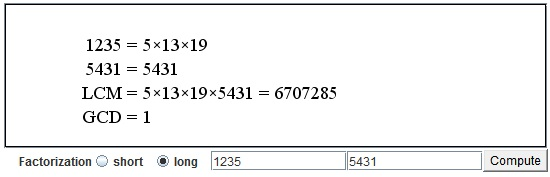# GCD and LCM by Plain Factorization

The applet below is an interactive illustration to the process of finding gcd and lcm of two integers by first finding the prime factorization of each. gcd stands for the "Greatest Common Divisor" and lcm for the "Least Common Multiple". Both can be found with the help of the factor tree. The process consists in comparing the exponents of every prime in the two given numbers. The prime becomes a factor of the lcm with the largest exponent and of the gcd with the smallest exponent. gcd and lcm of two integers are related by the formula

gcd(M, N) × lcm(M, N) = M × N.

It follows that to find one is sufficient to find another, for example, gcd(N, M) = N × M / lcm(N, M). gcd alone can be found with Euclid's algorithm; lcm of two or more numbers is produced by the onion algorithm.

In the applet, type two integers in the two fields at the bottom of the applet and press the Enter key or the "Compute" button. You can also add factors to the present numbers by continuing to type "*" followed by the desired factor. It is instructive to build the integers factor by factor. Try adding one factor at a time to one or both of the already present integers and see if you can figure out the effect this will have on gcd and lcm before pressing the "Compute" button".

(The applet can handle integers as large as 7,000,000,000. If you want to factor larger numbers have a look at Wolfram's Alpha.)

### If you are reading this, your browser is not set to run Java applets. Try IE11 or Safari and declare the site https:///www.cut-the-knot.org as trusted in the Java setup.What if applet does not run?• Factoring with the Factor Tree
• GCD and LCM via Factor Tree
• Common Multiples and the Least Common Multiple
• GCD(M, N) x LCM(M, N) = M x N
• Divisibility Criteria
• Euclid's Algorithm
• Algorithm for Computing the LCM
• Factors And Multiples
• Two Properties of Greatest Common Divisor
• Properties of GCD and LCM
• A Line in a Square Grid
• Number of Factors of an Integer
• Extension of Euclid's Game
•